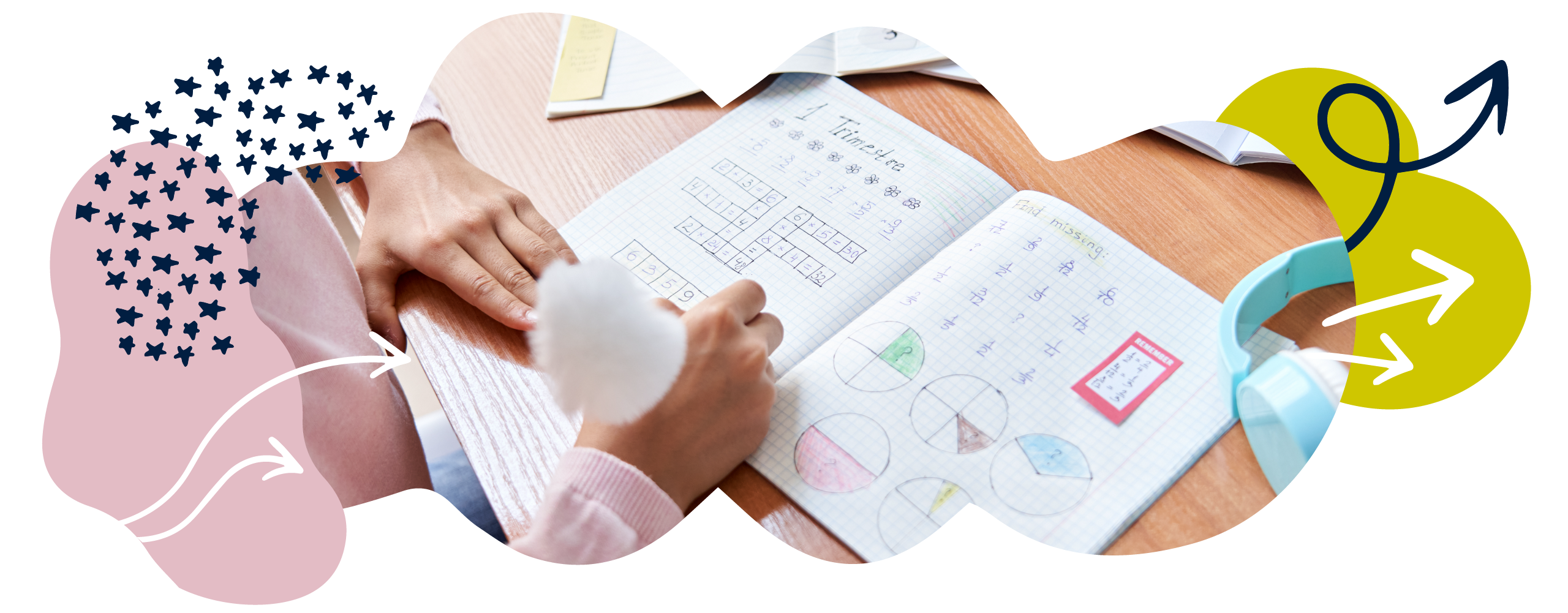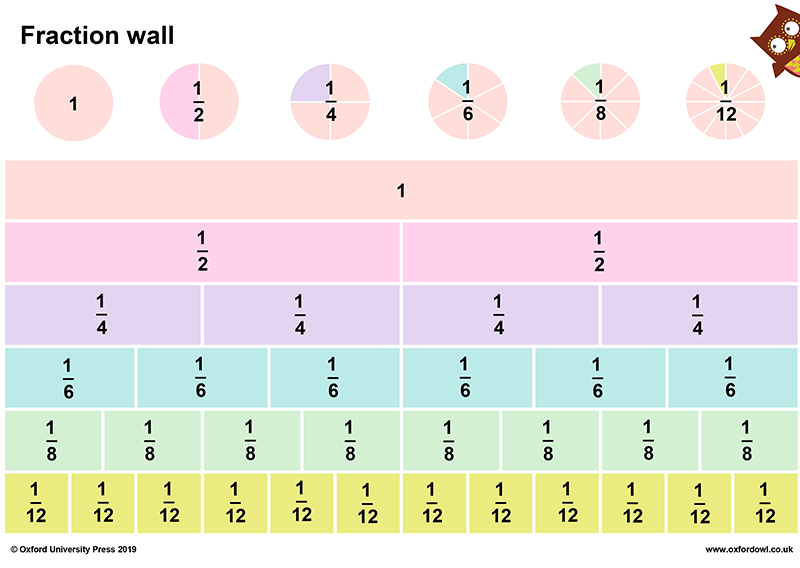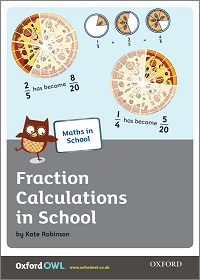We use cookies to enhance your experience on our website. By continuing to use our website, you are agreeing to our use of cookies. You can change your cookie settings at any time. Find out more# Fractions in Year 4 (age 8–9)

In Year 4, your child will explore the link between fractions and multiplication/division. They will also learn more about decimals, using numbers with up to two decimal places.

The key words for this section are non-unit fraction, denominator, numerator, factor, and place value.

## What your child will learn

Take a look at the National Curriculum expectations for fractions in Year 4 (ages 8–9):

#### Use diagrams to show equivalent fractions

In Year 4, your child will continue to explore equivalent fractions (for example is equivalent to ). As part of this, they will show families of common equivalent fractions using diagrams like fraction walls.

Your child will also learn to simplify fractions (for example =  or  = ).

#### Count up and down in hundredths

Your child will use their knowledge of place value and division to connect hundredths () to tenths ().  They will understand that hundredths can be created when dividing an object by 100, or by dividing tenths by 10.

They will also be expected to count up and down in hundredths mentally.

#### Solve problems involving quantities and non-unit fractions

Your child will calculate quantities involving fractions. For instance, they might be asked to find a fraction of a set of objects. They should be able to do this even when the answer is not a whole number. For example:

of 22 = 3

They will solve problems involving fractions to divide quantities, including non-unit fractions. For example:

of 12

First they will find the value of  = 4.

Then they will multiply this value by 2 to find  = 8.

#### Add and subtract fractions with the same denominator

Your child will use drawings, diagrams, and symbols to add and subtract fractions with the same denominator (the bottom number of a fraction). For example:#### Use decimal equivalents of tenths or hundredths

In Year 4, your child will learn that decimals and fractions are different ways of expressing numbers and proportions.

They will learn to find decimal equivalents of tenths or hundredths by dividing whole numbers by 10 and 100. For example, they will need to know that  = 0.1 and  = 0.01.

#### Use decimal equivalents of ¼, ½, ¾, and tenths and hundredths

Your child will learn to use decimal equivalents of any number of tenths or hundredths. For example:

is equivalent to 0.1.

is equivalent to 0.3.

is equivalent to 0.01.

is equivalent to 0.05.

is equivalent to 0.25.

is equivalent to 0.5.

is equivalent to 0.75.

#### Divide one- or two-digit numbers by 10 and 100

Your child will be able to divide numbers by 10 and 100.

They will know what happens when they divide a 1- or 2-digit number by 10 and 100, identifying the value of the digits in the answer as ones, tenths, and hundredths. For example:

3 ÷ 10 = 0·3 =

5 ÷ 100 = 0·05 =

#### Round numbers with 1 decimal place

Your child will round numbers with decimals up and down to the nearest whole number. For example:

1.6 will round up to 2.

18.3 will round down to 18.

#### Compare numbers with up to 2 decimal places

Your child will learn to compare decimal numbers with up to two decimal places and say which one is bigger or smaller. At this stage, they will mainly be comparing numbers with the same number of decimal places. For example:

3.45 is smaller than 3.54.

8.8 is bigger than 8.5.

#### Solve simple measure and money problems

Your child will solve real-world problems involving fractions and decimals to 2 decimal places.

They will also represent numbers with one or two decimal places in several ways, such as on number lines.

## How to help at home

There are lots of ways you can help your child to understand fractions. Here are just a few ideas.

### 1. Explore equivalent fractions

Equivalent fractions are different fractions that have the same value.

For example,  and  are equivalent.

You could help your child find equivalent fractions using fraction walls. For example, your child could make a fraction wall to work out that  is equivalent to , , , and so on.

### Fraction wallStick our fraction wall on the fridge!

Give your child a unit fraction, such as  , and ask them to find as many equivalent fractions as they can. See if they can write down the equivalent fractions they find, such as  =  = . Why not try against the clock?

When they have done this, ask them if they notice any patterns in the sequence of fractions. Help your child notice that you can change one fraction into an equivalent fraction by multiplying (or dividing) the numerator and the denominator by the same number.

### 2. Simplify fractions

Your child will learn to simplify fractions. Simplifying a fraction means reducing the fraction into its simplest form.

To simplify fractions, your child will find the highest common factor of the numerator and the denominator and then divide both numbers by it. The ‘highest common factor’ is the biggest number that can be divided equally into both the numerator and the denominator. For example:

Simplify .

We know that 5 is the highest common factor of 5 and 25 (i.e. it is the biggest number that both 5 and 25 can be divided by to arrive at whole numbers).

We can therefore divide the numerator and the denominator by 5 to get the answer: .

If the numerator and the denominator do not have a common factor, then they are fully simplified. See page three of our Fraction Calculations in School booklet for more information.

### Fraction Calculations in SchoolFind out how your child is taught to calculate with fractions in school.

### 3. Think about fractions while shopping

Shopping together can provide a useful opportunity to think about fractions as decimals.

Encourage your child to compare price tags. This is great practice for comparing numbers with two decimal places. For example, one item may cost £1.67 and another may cost £1.76. Ask your child to say which one is bigger and to explain how they know. They could even use the greater than > or less than < symbols to compare numbers up to two decimal places (for example, 5.67 < 5.71).

Try using place value charts to help your child compare numbers with two decimal places. Place value charts are a way of showing how big numbers are visually. For example:By showing the numbers with a place value chart, your child can understand the value of the number more easily. For example, place value charts could help with this comparison:

4.20 has four ones, two tenths and zero hundredths.

4.76 has four ones, seven tenths and six hundredths.

Therefore, 4.76 is greater than 4.20.

### 4. Round decimals

Your child will need to be able to round decimals with one decimal place to the nearest whole number.

When you are shopping, ask your child to round prices to the nearest pound. For example, if an item costs £4.70, ask them to round it up to the nearest pound (£5). The same could be done to work out approximately how much change you might expect.